# Standard Costing: Easy and Simple way to learn Formula

Most of the students thought that the topic STANDARD COSTING is very time consuming and have covered only 5-10 marks in Exams, so chal yr leave it, itne me to koi aur topic cover kr lege. Actually the problem is not that we can’t understand the concepts of this chapter, but the issue is to retain all the formula. So, it’s like that Samaj to sb aa gya yr pr yaad kaise hoga itna sara.

So, today in this article, I am going to share a trick to learn all the formulas and you will see that it will help us to solve all the questions easily. Trust me this topic is not tough. It is very easy and simple.

So let’s start….

Very Firstly, we have to understand what are the terms based on which we have to learn formulas….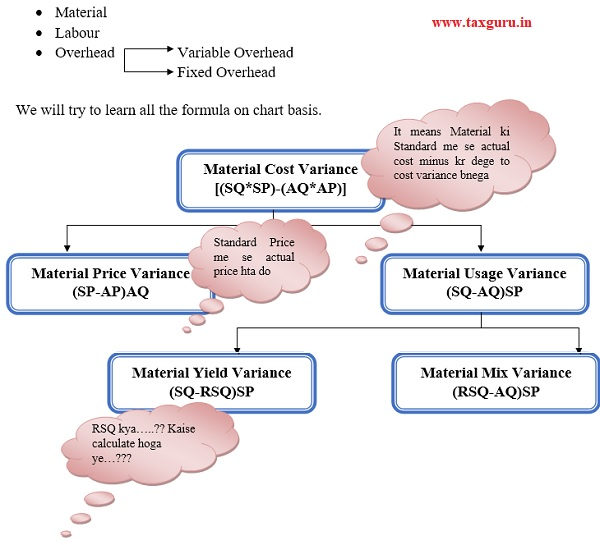SQ Standard Quantity for actual output

AQ  Actual Quantity Used

SP    Standard Price per Kg

AP Actual Price per kg

RSQ  Revised Standard Quantity used

It Means Total to lelo AQ wala and usko apportion krdo in ratio of SQ

EXAMPLE:

 Product SQ AQ RSQ A 50 60 70 B 50 80 70 TOTAL 100 140 140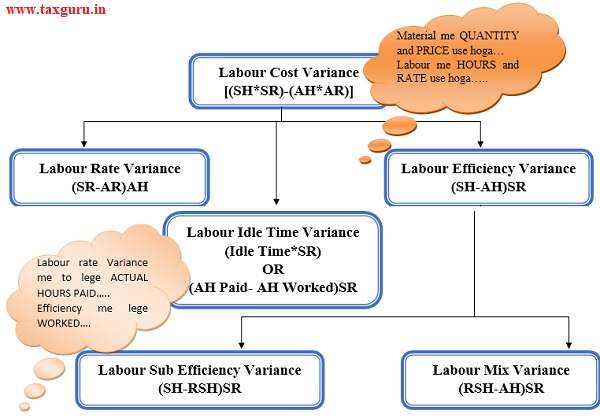SH Standard Hours for actual output

AH Actual Hours

SR Standard Rate per Hour

AR  Actual Rate per Hour

RSH Revised Standard Hours

It Means Total to lelo AH wala and usko apportion krdo in ratio of SH.

EXAMPLE:

 Product SH AH RSH A 50 60 70 B 50 80 70 TOTAL 100 140 140

NOTE: RSH is also calculated in same manner as we calculate RSQ.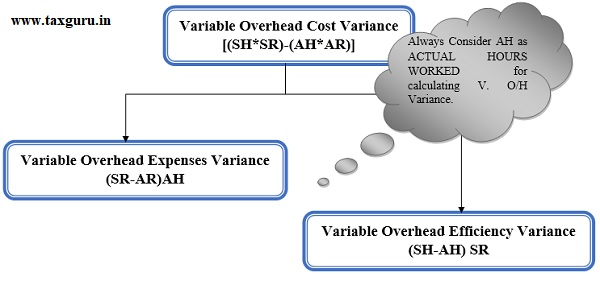NOTE: Variable Overhead Expenses Variance is also known as RATE VARIANCE OR SPENDING VARIANCE.

Lastly, Going to discuss Fixed Overhead variance, the toughest range of formula. But I will say again, you will memories easily.

To learn Fixed Overhead, Firstly calculate FIVE items.

1. Standard Hours for Actual Output * Standard Fixed Overhead Per Hour

2. Actual Hours * Standard Fixed Overhead Per Hour

3. Standard Hours for Actual days * Standard Fixed Overhead Per Hour

4. Budgeted Fixed Overhead (Budgeted Hours * Standard Fixed Overhead Per Hour)

5. Actual Fixed Overhead

NOTE: Calculate these FIVE items in order as mentioned above.

Then, Draw a chart to arrange all parts and calculate variance according to the numbering given.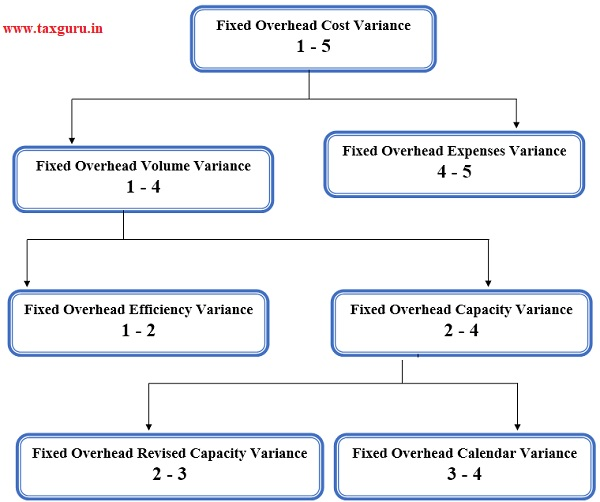For exams Perspectives, chart always remains easiest form to retain all the formula properly.

In Fixed Variance, we have to calculate FIVE items firstly and then according to chart we can calculate all the variances correctly. We have to deduct the values as mentioned above in the chart according to numbering.

EXAMPLE:

If we have calculated the FIVE items as per the details given in the question and the values are:

1. SH * SR   2250/-

2. AH * SR 2200/-

3. Standard Hours for Actual days * SR 1900/-

4. BH * SR 2000/-

5. Actual Overhead 2000/-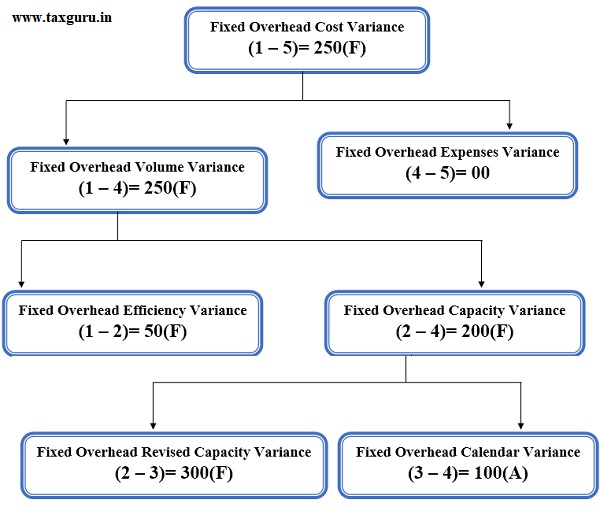#### Author Bio

Qualification: CA in Practice
Company: N/A
Location: Meerut, Uttar Pradesh, India
Member Since: 21 Apr 2021 | Total Posts: 2

June 2021
M T W T F S S
123456
78910111213
14151617181920
21222324252627
282930